# Multiplexers: How Do They Work? (Circuit of 2 to 1, 4 to 1, 8 to 1 MUX)

Contents

## What is a Multiplexer?

A multiplexer (sometimes spelled multiplexor and also known as a MUX) is defined as a combinational circuit that selects one of several data inputs and forwards it to the output. The inputs to a multiplexer can be analog or digital. Multiplexers are also known as data selectors.

A multiplexer is useful for transmitting a large amount of data over the network within a certain amount of time and bandwidth.

Multiplexers that are built from transistors and relays are termed as analog multiplexers which are used in analog applications and Multiplexers that are built from logic gate termed as digital multiplexers which are used in digital applications. The inverse of a multiplexer is known as a demultiplexer.

## What Does a Multiplexer Do?

In digital systems, many times it is necessary to select a single data line from several data-input lines and the data from the selected data input line should be available on the output line. The digital circuit which does this task is a multiplexer.

A multiplexer is a digital circuit that selects one of the n data inputs and forwards it to the output. The selection of one of the n inputs is done by the select inputs. To select one of several inputs, we need m select lines such that 2m=n.

Depending on the digital code applied at the select inputs, one of the n data inputs is selected and transmitted to the single output. Hence, a multiplexer has maximum 2n data input lines, ‘m’ selects lines, and one output line.

The block diagram of an n-to-1 multiplexer and its equivalent circuit is shown in the figure below.

## How Does a Multiplexer Work?

The multiplexer works like a multiple-input and single-output switch. The output gets connected to only one of the n data inputs at a given instant of time. Therefore, the multiplexer is ‘many into one’ and it works as the digital equivalent of an analog selector switch.

## Multiplexer Circuit

There are many types of multiplexers – like 2-to-1, 4-to-1, and 8-to-1 multiplexers. Each one has a different circuit, truth table, boolean expression, and working principle. Let’s discuss each type of multiplexer one by one.

### 2 to 1 Multiplexer

#### 2 to 1 Multiplexer Circuit

A 2-to-1 multiplexer is the digital multiplexer circuit that has two data inputs D0 and D1, one selects line S and one output Y. To implement a 2-to-1 multiplexer circuit we need 2 AND gates, an OR gate, and a NOT gate.

The block diagram, logic symbol and switching circuit analogy of 2-to-1 multiplexer is shown in the figure below.

As shown, D0 is an applied input to one of the AND gate and D1 is an applied input to the other AND gate. Select input S is applied to the second AND gate as a second input and an inverted input S is applied to the first AND gate as a second input. The output of both the AND gates is applied as inputs to the OR gate.2 to 1 Multiplexer Circuit

#### 2 to 1 Multiplexer Working Principle

When S=0, it is applied directly as an input to the second AND gate, and inverted S that is 1 is applied as a second input to the first AND gate. Now, we know that for AND gate if any one input is zero, the output is zero. So, the output of the second AND gate is zero. Since the second input to the first AND gate is 1, its output is equal to its first input, which is Y = D0.

When S=1, Exactly the reverse happens. In this case, the second AND gate output is equal to its first input, that is Y = D1 and the first AND gate output is 0.

So, by applying either a logic ‘0’ or a logic ‘1’ at the select input S, we can select the appropriate input, D0 or D1 with the circuit act like a single pole double throw (SPDT) switch.

#### 2 to 1 Multiplexer Truth Table

The below table shows the truth table for the 2-to-1 multiplexer.

Here, the 2-input multiplexer connects one of two 1-bit sources to a common output, hence it produces a 2-to-1 multiplexer.

#### 2 to 1 Multiplexer Boolean Expression

From the truth table, we can write the boolean expression for the output of the 2-to-1 multiplexer.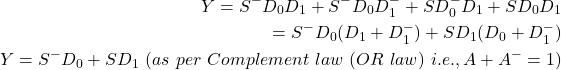In simple notation

As shown in the above table that when select input S=0 then output Y=D0 and when S=1 then Y=D1. We can increase the number of data inputs to be selected further and larger multiplexer circuits can be implemented using smaller 2-to-1 multiplexer.

We can also implement all the multiplexers by using the NAND gate. Note that NAND and NOR gates are a universal gate and we can implement any digital circuits by using NAND and NOR gates.

### 4 to 1 Multiplexer

#### 4 to 1 Multiplexer Circuit Diagram

A 4-to-1 multiplexer is a digital multiplexer that has four data inputs, two select lines, and one output. To implement a 4-to-1 multiplexer circuit we need 4 AND gates, an OR gate, and a 2 NOT gate.

In a 4-to-1 multiplexer, four inputs D0, D1, D2, and D3, two data select lines that are S0 and S1 as 4-inputs represent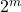=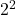=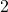data control lines. One of these four inputs will be connected to the output based on the combination of the inputs at the selection lines.

The block diagram, logic symbol and switching circuit analogy of 4-to-1 multiplexer is shown in the figure below.

#### 4 to 1 Multiplexer Working Principle

If both the select inputs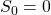,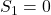then the topmost AND gate is enabled and all other AND gate is disabled. So, the data input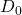is selected and transmitted as output. Hence, we get the output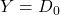.

If both the select inputs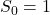,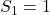then the bottom-most AND gate is enabled and all other AND gate is disabled. So, the data input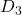is selected and transmitted as output. Hence, we get output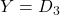.

#### 4 to 1 Multiplexer Truth Table

The below table shows the truth table for the 4-to-1 multiplexer.

Here, the 4-input multiplexer connects one of four 1-bit sources to a common output, hence it produces a 4-to-1 multiplexer.

#### 4 to 1 Multiplexer Boolean Expression

From the truth table, we can write the Boolean expression for the output.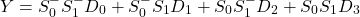### 8 to 1 Multiplexer

#### 8 to 1 Multiplexer Circuit Diagram

An 8-to-1 multiplexer is a digital multiplexer that has 8 data inputs, 3 select lines, and one output. To implement an 8-to-1 multiplexer circuit we need 8 AND gates, an OR gate, and a 3 NOT gate.

In 8-to-1 multiplexer, eight inputs D0, D1, D2, D3, D4, D5, D6, and D7, three data select lines that are S0, S1, and S2 as 8-inputs represent 2m = 23 data select lines. In an 8-to-1 multiplexer, the selection of a particular input line is controlled by three selection lines.

The block diagram of the 8-to-1 multiplexer is shown in the figure below.

#### 8 to 1 Multiplexer Working Principle

If all the three select inputs,,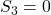then the topmost AND gate is enabled and all other AND gate is disabled. So, the data input D0 is selected and transmitted as output. Hence, we get the output.

If all the three select inputs,,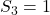then the bottom-most AND gate is enabled and all other AND gate is disabled. So, the data input D7 is selected and transmitted as output. Hence, we get output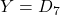.

#### 8 to 1 Multiplexer Truth Table

The below table shows the truth table for the 8-to-1 multiplexer.

#### 8 to 1 Multiplexer Boolean Expression

From the truth table, we can write the Boolean Expression for the output.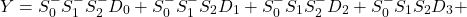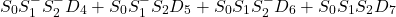### Arduino Multiplexer

Multiplexing is a very efficient technique for controlling many components wired together in the form of an array or matrix – and this holds true for Arduino. Such components are LEDs, sensors, buttons, and other components that come included in any of the best Arduino starter kits.

The configuration of the Multiplexer IC to Arduino is shown in the figure below. Here, we used the 8-channel 74HC4051 multiplexer IC.

Configuration of Multiplexer IC 74HC4051 to Arduino:

• +VCC supplies to the IC and it is connected to the 5V supply pin of Arduino.
•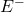is the enable pin, -VEE is the negative supply voltage pin and the GND pin is connected to the GND pin of Arduino.(Note thatis generally active low terminal, it means that it will enable the chip when it is connected to GND. If we connect it to 5V supply, it will disable the multiplexer chip and gives output Y=0 irrespective of any input condition.)
• The control pins S0, S1, and S3 controls the output and it is connected to the 2, 3, and 4 pins of Arduino.
• The output Y is connected to any of the digital input pins 6 to 12 of Arduino if inputs to the chip is digital. But if the inputs to the chip is analog then the output pin is connected to the analog input pins of A0 to A5 of Arduino.

Here, inputs to the multiplexer can be sensors, buttons, or potentiometers. Note that input to the multiplexers can either be analog or digital. Some people prefer to use Raspberry Pis instead of Arduinos. If you’re unsure, you may want to learn about Arduino vs Raspberry Pi.

## Multiplexer Integrated Circuits (ICs)

The available multiplexers integrated circuits (ICs), their functions, and the output state is listed in the below table.

## What is a Multiplexer Tree or Chaining Multiplexers?

The multiplexers having a greater number of inputs can be obtained by cascading two or more multiplexers with a lesser number of inputs.

In other words, larger multiplexers can be constructed by chaining together of smaller multiplexers. This is known as a multiplexer tree or a chaining multiplexer.

For example, an 8-to-1 multiplexer can be constructed by cascading two 4-to-1 and one 2-to-1 multiplexer. The output of the two 4-to-1 multiplexers is given to the 2-to-1 multiplexer with the select lines on the 4-to-1 multiplexers put in parallel that gives a total number of select inputs to 3, which is equivalent to an 8-to-1 multiplexer. Lets see one example.

### Example: Obtain an 8-to-1 Multiplexer Using Two 4-to-1 Multiplexers

Solution:

• The cascading of two 4-to-1 multiplexer results in the 8-to-1 multiplexer as shown in the figure below.
• There are 8 data inputs that are D0 to D7.
• The select inputs S0 and S1 of both the 4-to-1 multiplexers are connected in parallel whereas the third select input S2 is used for enabling one multiplexer at a time.
• Select input S2 is connected directly to the Enable(E) terminal of MUX-1 whereas S2 is connected to the enable terminal of MUX-2.
• The output of both the multiplexers is connected to the OR gate to obtain the final output Y.

The truth table is shown in the below table. We get the output,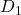,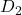, andwhen MUX-1 is enabled and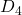,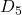,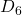, and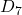when MUX-2 enabled.

Some of the advantages of a Multiplexer include:

• A multiplexer reduces the number of wires used. Hence it reduces the circuit complexity and overall cost.
• A multiplexer improves the reliability of the digital systems because it reduces the number of external wired connections.
• We can implement many combinational circuits using MUX.
• Multiplexer simplifies the logic design.
• Multiplexer does not need the k-maps (Karnaugh map) and simplification.

## Applications of a Multiplexer

Some of the applications of a Multiplexers include:

Communication Systems

• Multiplexers are used as a data selector to select one out of many data inputs in communication systems to transmit the various types of data (audio, video, etc.) at the same instant. Hence it increases the efficiency of the communication system by allowing various types of data into single transmission lines.

Telephone Networks

• In a telephone network, multiplexers can be used to transmit multiple audio signals into a single channel.

Computer Memorys

• In computer memory, multiplexers are used to implement a large amount of data, at the same instant reduces the number of copper wires required to connect memory to other parts in the computer.

Transmission from the computer system to a satellites

• Multiplexers are also used to transmit the data from the computer system of a spacecraft or satellite to the earth by utilizing “GPS” (Global Positioning System) and “GSM” (Global System for Mobile Communication).

Some other applications include:

• Multiplexers can be used to implement the combinational logic circuit like time-multiplexing systems and frequency multiplexing systems, A/D and D/A converter.
• Multiplexers can be used to implement Boolean functions of multiple variables.
• Multiplexers are used in data acquisition systems.

Want To Learn Faster? 🎓
Get electrical articles delivered to your inbox every week.
No credit card required—it’s 100% free.